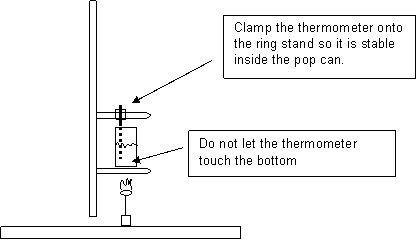CHEMISTRY 1 – CALORIMETRY LAB                 NAME                                                                           HOUR

OBJECTIVE

Determine the energy content (in calories) of a food product

Materials:

Walnut, stopper with wire, pop can, temperature probe/computer, ring stand, ceramic centered wire gauze, clamps

PROCEDURE/RATIONALEBurn the food, and use the heat generated to heat a measured quantity of water using the temperature probe from the computer. Run the Logger Pro file “Calorimetry Lab” in the Chemistry 1 folder on the desktop.

Measure the temperature of the water to determine the temperature change.

Use the equation Q = s x m x DT to determine the energy content of the food.

LAB DATA                                                                    PACKAGE DATA

Mass of food                                        g                     Type of food

Volume of water in calorimeter               mL                   Serving size                              g

Initial Temperature of water                    oC                    Calories per serving                   Cal =                 kcal

Final Temperature of water                     oC                    (Note:  Calories listed in nutritional info are actually kcal)

CALCULATIONS

Note:    s = 1.0 cal/goC; 1 cal = 4.184 J, 1 mL H2O = 1 g H2O

Mass of Water in Calorimeter

Temperature Change (DT ) of Water

In calories:

In Joules:

Energy content (J/g) of the food

Calculate the energy content (J/g) that would be expected, based upon the data given on the food package.  Compare this with the energy content that you measured in the lab.  Comment on the variations you observe. (Help)

 1 serving kcal cal J g serving kcal cal